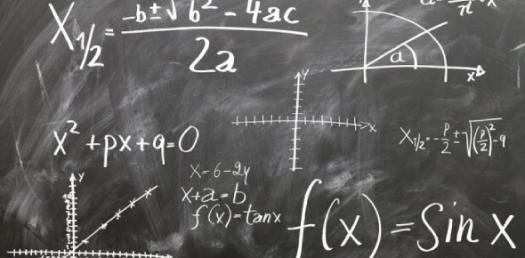# Algebra 1 Quiz With Answers

10 Questions | Attempts: 10189SettingsDo you think you have a good understanding of algebra? Check out this awesome algebra 1 quiz that we have created below for you. Algebra is an interesting and bit tricky topic of mathematics subject. Will you be able to pass this test? Well, try taking this quiz, and you can find out the answer today itself! So, give this quiz a try and get to test your knowledge. Ready? Let's start then.

• 1.
42  =
• A.

8

• B.

16

• C.

6

• D.

1

• 2.
What will be the value of x in this equation-  2x - 4 = 0
• A.

0

• B.

-2

• C.

2

• D.

1

• E.

4

• 3.
Use the formula above, when x = -1to find the value for y.
• A.

6

• B.

-4

• C.

8

• D.

5

• E.

0

• 4.
What is the slope of a line containing the points (3,2) and (-1,0).
• A.

-3/2

• B.

-1

• C.

2/1

• D.

1/2

• 5.
Solve: 4/2 + 7/2 =
• A.

5 1/4

• B.

5 1/2

• C.

11/4

• D.

7/4

• E.

5 2/1

• 6.
Use the formula above to find a when b = 4, c = 5.
• A.

2

• B.

1

• C.

4

• D.

6

• E.

3

• 7.
Find the value of x in this equation- x + 2(x + 7) = 14x– 7.
• A.

2

• B.

3

• C.

6

• D.

5

• 8.
What will be the value of z in this equation- 5(z + 1) = 3(z + 2) + 11.
• A.

6

• B.

-6

• C.

4

• D.

9

• 9.
(x – 2) / 4 – (3x + 5) / 7 = – 3, x = ?
• A.

5

• B.

15

• C.

10

• D.

2

• 10.
12t – 10 = 14q + 2. Find q.
• A.

8

• B.

6

• C.

-6

• D.

0

## Related TopicsBack to top
×

Wait!
Here's an interesting quiz for you.